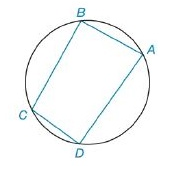Chapter 8.2, Problem 12E### Elementary Geometry for College St...

6th Edition
Daniel C. Alexander + 1 other
ISBN: 9781285195698

#### Solutions

Chapter
Section### Elementary Geometry for College St...

6th Edition
Daniel C. Alexander + 1 other
ISBN: 9781285195698
Textbook Problem
7 views

# For Exercises 11 and 12, use Brahmagupta’s Formula.For cyclic quadrilateral A B C D , find the area if A B   =   6 cm, B C   =   7 cm, C D   =   2 cm, and D A   =   9 cm.To determine

To Find:

The area of a cyclic quadrilateral using Brahmagupta’s Formula.

Explanation

Formula Used:

For a cyclic quadrilateral with sides of lengths a,b,c and d, the area A is given by

A=(s-a)(s-b)(s-c)(s-d),

where s=12(a+b+c+d).

Calculation:

Consider, AB=a=6 cm,BC=b=7 cm CD=c=2 cm andAD=d=9 cm.

s=126+7+2+9=12

### Still sussing out bartleby?

Check out a sample textbook solution.

See a sample solution

#### The Solution to Your Study Problems

Bartleby provides explanations to thousands of textbook problems written by our experts, many with advanced degrees!

Get Started